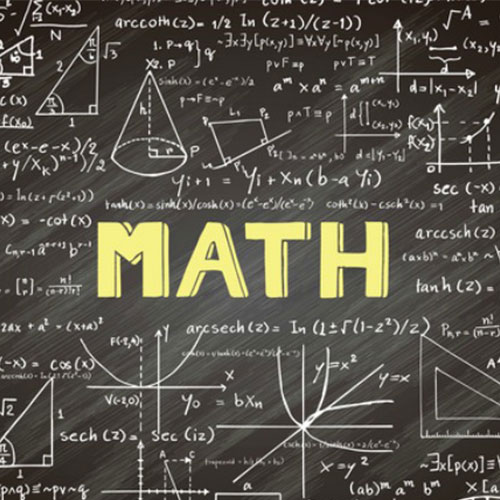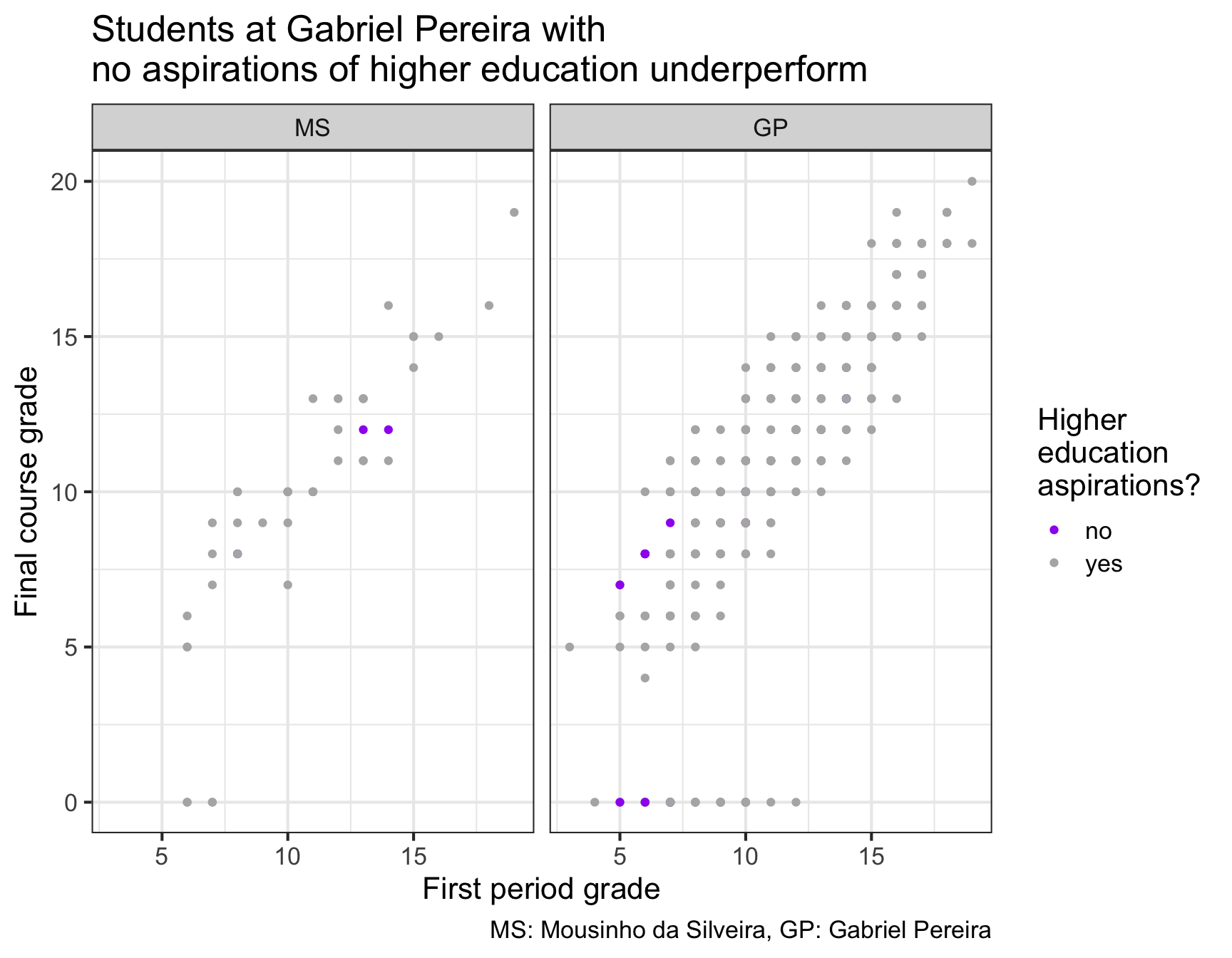# HW 03 - Student Math Performance

### Due: Thursday, Mar 05 at 11:59pmThis homework is based on student math achievement in secondary education of two Portuguese schools. The data include student grades, demographic, social and school related features. Our objective in this analysis is to understand how some of these variables affect students’ final grade. Data was collected by using school reports and questionnaires.

Source: Paulo Cortez, University of Minho, GuimarÃ£es, Portugal, http://www3.dsi.uminho.pt/pcortez

# Getting Started

Navigate to your repository beginning with hw3- and clone it into RStudio Cloud. Configure git by using the use_git_config() function in the usethis package, and finally, give your project a meaningful name (hw3-[name]). You may cache your login credentials, but remember they are only stored for this single project.

Be sure to follow good coding style and commit often.

# Packages and data

In addition to lm(), c(), and factor(), your code should only contain functions from the loaded R packages below unless explicitly stated in an Exercise.

library(tidyverse)
library(broom)

The data is in a csv file in folder data of your repository. Read it in to R with

math <- read_csv("data/math_performance.csv")

A data dictionary is also included in the data folder. You’ll want to consult this to understand the variable definitions.

# Exercises

## Data wrangling

1. For variables that R thinks are numeric but are actually categorical, set them to be factors. Overwrite the original data frame and variables.

2. For variables that are of type character, set them to be factors. Overwrite the original data frame and variables. You may use is.character().

3. Relevel variable school so Mousinho da Silveira is set as the baseline level. Overwrite the original data frame and variable.

## EDA

1. Recreate the visualization below. Set the figure width and height to be 9 and 7, respectively. Some hints are given further below the plot.Hints:

• colors: purple, grey70
• theme: bw
• base font size: 16
1. What is the final grade failure rate at each school? Assume a final grade of 0 is failure. Your output should only included the necessary variables to answer the question.

2. Based on your analysis in Exercise 5, what is deceiving about the plot in Exercise 4?

3. Create a single visualization to explore the relationship between g3 and at least two other variables in the dataset. At least one of the two variables should be a categorical variable. You may not recreate a variation of the plot in Exercise 4. As always, be sure to follow best visualization guidelines.

## Modeling

1. Subset math so it only contains information on students that passed the course. Save the resulting data frame with a meaningful name (do not overwrite math). Use this subsetted data frame going forward.

2. Fit a linear model to explore how the first period grade and school are associated with the final course grade. Write out the linear model, interpret the coefficients in context of the data, and determine and interpret $$R^2$$. Do not include interactions.

3. Fit a linear model to explore how the first period grade, school, and family size are associated with the final course grade. Write out the linear model, interpret the coefficients in context of the data, and determine and interpret $$R^2$$. Do not include interactions.

4. Compare $$R^2$$ and adjusted $$R^2$$ for the models you fit in Exercises 9 and 10. What do you observe? Explain why this happened. Hint: use pull() to show the metric as a vector and it will automatically print more digits after the decimal.

5. Fit a linear model to explore how the first period grade and school are associated with the final course grade. You should include interactions. Write out the linear model.

6. Consider the following full model, where we explore how first period grade, absences, school, sex, age, internet, and parent’s status affect the final course grade. Perform backward elimination using AIC as the criterion with function step(). You do not need to consider interactions. Output the model result in a tidy data frame format.

7. Interpret the coefficients of two variables in your final model from backward elimination.

8. Is the linearity assumption satisfied for the final model in Exercise 13? Create an appropriate diagnostic plot and describe what you observe. As always, be sure to follow best visualization guidelines.

9. Three other students did not receive a first period grade. Some of their information is below.

• Student A: age - 18, sex - F, school - GP, internet - yes, pstatus - T, absences - 12
• Student B: age - 17, sex - F, school - MS, internet - yes, pstatus - A, absences - 11
• Student C: age - 18, sex - M, school - MS, internet - no, pstatus - A, absences - 23

Suppose the instructor imputes their missing first period grade based on the median first period grade from their respective school. Using this information and your final model fit in Exercise 13, predict each student’s final grade. Be sure to use the permitted functions.

# Submission

Knit to PDF to create a PDF document. Stage and commit all remaining changes, and push your work to GitHub. Make sure all files are updated on your GitHub repo.

Please only upload your PDF document to Gradescope. Before you submit the uploaded document, mark where each answer is to the exercises. If any answer spans multiple pages, then mark all pages. Make sure to associate the “Overall” section with the first page.